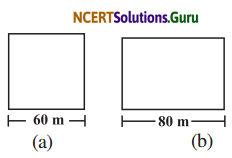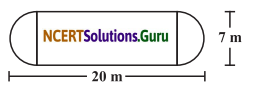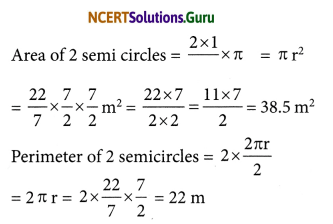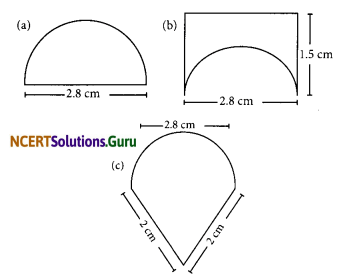# NCERT Solutions for Class 8 Maths Chapter 11 Mensuration Ex 11.1

These NCERT Solutions for Class 8 Maths Chapter 11 Mensuration Ex 11.1 Questions and Answers are prepared by our highly skilled subject experts.

## NCERT Solutions for Class 8 Maths Chapter 11 Mensuration Exercise 11.1

Question 1.
A square and a rectangular field with measurements as given in the figure have the same perimeter. Which field has a larger area?(a) Side of the square = 60 m.
∴ Perimeter of the square = 4 × side
= 4 × 60 = 240 m
Area of the square = side × side
= 60 × 60 m2 = 3600 m2

(b) Length of the rectangle = 80 m
Perimeter of the rectangle = Perimeter of a square
2 (l + b) = 240
2 (80 + b) = 240
160 + 2b = 240
2b = 240 – 160 = 80 ,
b = $$\frac { 80 }{ 2 }$$ = 40 m
Area of the rectangle = l × b = 80 × 40m2
= 3200 m2
3600 m2 > 3200 m2
∴ Area of the square field is greater.Question 2.
Mrs Kaushik has a square plot with the measurement as shown in the figure. She wants to construct a house in the middle of the plot. A garden is developed around the house. Find the total cost of developing a garden around the house at the rate of ₹ 55 per m2.Side of a square = 25 m
Area of the plot = side × side = 25 × 25 m2 = 625 m2

In the given diagram, the construction portion is a rectangle with length = 20 m and breadth = 15 m

Area of the construction portion = Area of a rectangle = 20 × 15 m2 = 300 m2

Area of the garden = (625 – 300) m2 = 325 m2 Cost of developing the garden = ₹ 55 × 325 = ₹ 17,875

Question 3.
The shape of a garden is rectangular in the middle and semi circular at the ends as shown in the diagram. Find the area and the perimeter of this garden (Length of rectangle is 20 – (3.5 + 3.5) metres].Radius of the semicircles = $$\frac { 7 }{ 2 }$$Length of the rectangle = 20 – (3.5 + 3.5) m = 20 – 7 = 13 m
Breadth of the rectangle = 7m
Area of the rectangle = l × b = 13 × 7 = 91m2
Perimeter of the rectangle = 2 (l + 0) (Breadth will not be consider)
= 2 (13 + 0) m = 2 × 13 = 26 m
Area of the garden = 38.5 + 91m2 = 129.5 m2
Perimeter of the garden = 22 + 26 m = 48 mQuestion 4.
A flooring tile has the shape of a parallelogram whose base is 24 cm and the corresponding height is 10 cm. How many such tiles are required to cover a floor of area 1080 m2? (If required you can split the tiles in whatever way you want to fill up the corners).
Base of the parallelogram = 24 cm = $$\frac{24}{100}$$ m
Corresponding height = 10 cm = $$\frac{100}{10}$$
Area of a tile = base × height
= $$\frac{24}{100} \times \frac{10}{100}$$ m2 = $$\frac{24}{1000}$$ m2
Area of the floor = 1080 m2
Number of tiles = $$\frac{\text { Area of the floor }}{\text { Area of one tile }}$$
= $$\frac{1080 \times 1000}{24}$$ = 45 × 1000 = 45000 tiles

Question 5.
An ant is moving around a few food pieces of different shapes scattered on the floor. For which food-piece would the ant have to take a longer round? Remember, circumference of a circle can be obtained by using the expression c = 2πr, where r is the radius of the circle.(a) Radius of the semicircle = $$\frac{2.8}{2}$$ = 1.4 cm
Perimeter of the semicircle = $$\frac{2 \pi \mathrm{r}}{2}$$ + 2r
= $$\frac{22}{7}$$ × 1.4 + 2 × 1.4 = 4.4 + 2.8cm
= 7.2 cm

(b) Perimeter of the semicircular part
= $$\frac{1}{2}$$ × 2πr = πr = $$\frac{22}{7}$$ × 1.4 = 4.4 cm
Perimeter of the rectangular part
= 1.5 + 2.8 + 15 cm = 5.8 cm
Perimeter of the given diagram
= 4.4 cm + 5.8 cm = 10.2 cm

(c) Perimeter of the semicircular part
= $$\frac{2 \pi r}{2}$$ πr = πr = $$\frac{22}{7}$$ × 1.4 cm = 4.4 cm
Perimeter of the given diagram
= (4.4 + 2 + 2) cm = 8.4 cm
∴ 7.2 cm < 8.4cm < 10.2 cm
Ant has to take a longer round for (b).

error: Content is protected !!